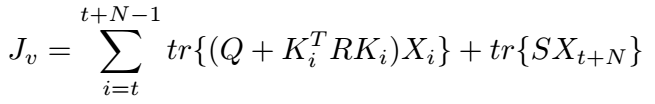# How to implement following objective function by using cvx?

How to implement following objective function by using cvx?where tr(~) denote trace of matrix.

What is the input data? What are the CVX (optimization) variables or expressions?

After you are clear on that, then you should determine the convexity or not of the objective function. If convex, then what are you having difficulty in implementing?

If everything is input data, except for the X_i 's, you should be able to build up the objective function using a for loop to handle the summation.

``````Objective = 0
for i = t:t+N-1
Objective = Objective + <current term>
end
minimize(Objective)
``````

Edit: if each X_i is a matrix, then declare `X` as a 3-D array, and use `X(:,:,i)` for X_i.

Thank you for you advises. I am very sorry for not explaining clearly the problem formulation.

Actually, the problem formulation is

My questions lie in two aspect:

1. As shown in picture, the decision variable is a sequence of matrix, so how to define them in script when we optimize? Using routine cell to define them or routine variables to define them??
2. Maybe I can build up the objective function using a for loop to handle the summation, but is this for loop outside of the cvx_begin or inside of the cvx_begin? Does it effect?

Thanks.

1. Use a 3D array to declare a sequence of matrix variables:
`variable K(m,m,N)`

2. The for loop must be after (“inside”) cvx_begin and variable declarations, and before the minimize statement.

Your serious problem is that if K is the decision variable sequence, the problem is not convex, and can not be handled by CVX. I will assume hte problem is non-convex, unless and until you prove otherwise.Why isn't CVX accepting my model? READ THIS FIRST!